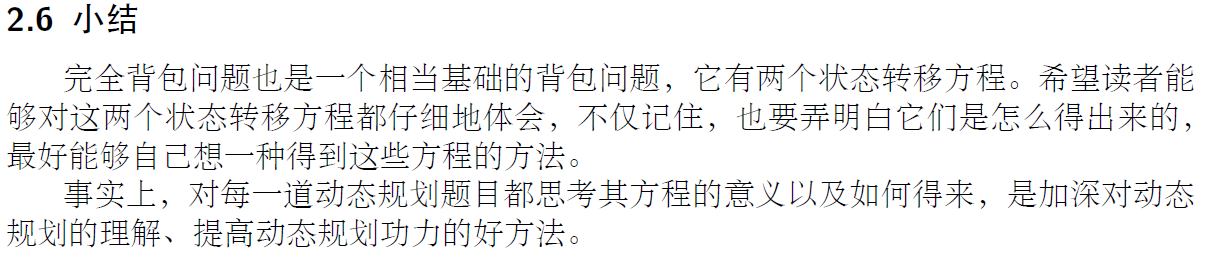# 动态规划的背包问题，看完这篇博客，你再不会就来打我——背包九讲详细学习笔记（目前已更新完0/1背包，完全背包部分一周内更新）

## 前言：

《背包九讲》的作者为崔添翼（dd_engi），原文链接不知道在哪里……这篇文章记录了我自己阅读大佬文章时不理解的地方是如何去思考的。并且给初学者提供了学习参考。

## 1、0/1背包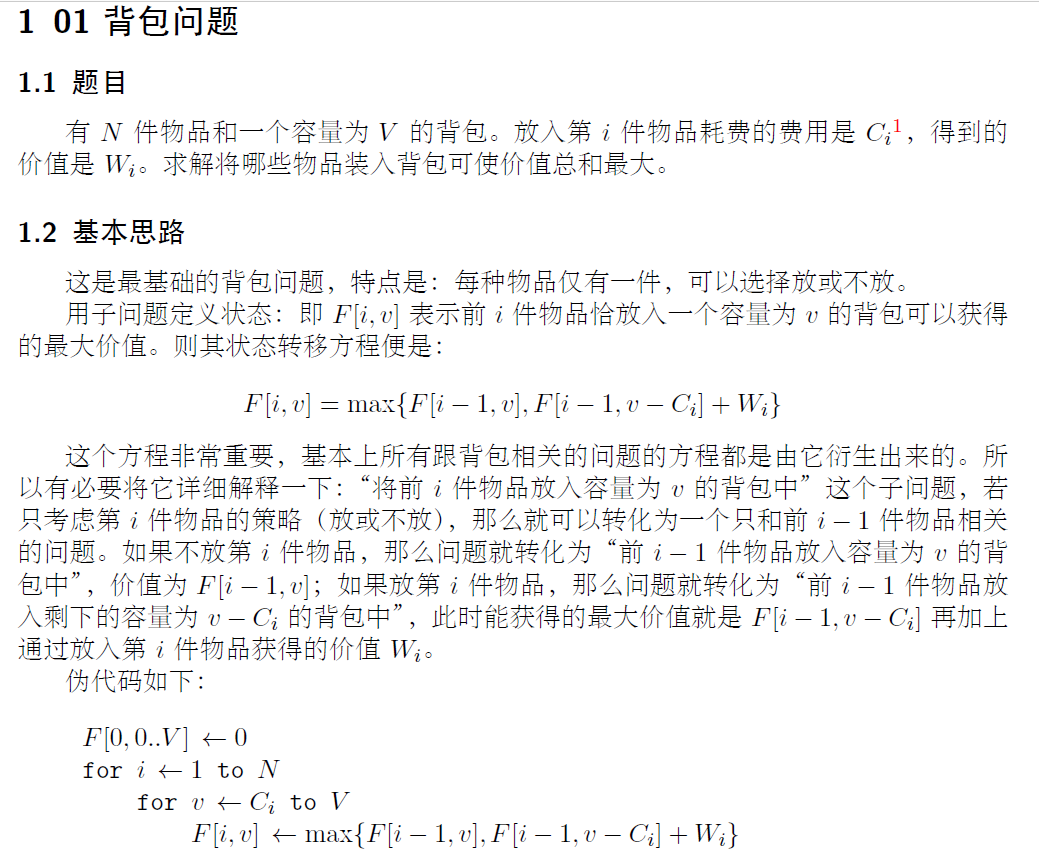### 最直观的输出过程理解：

``/*** 前提条件：所有的物品重量都为 1* @param things 物品列表* @param space 背包容量* @param fiv 那个博主巨好奇的状态转移中间的二维数组*/public static int method1(int[] things, int space, int[][] fiv) {for (int i = 0; i < things.length; i++) {for (int v = 1; v <= space; v++) {if (i == 0) {fiv[i][v] = things[i];} else {fiv[i][v] = Math.max(fiv[i - 1][v], fiv[i - 1][v - 1] + things[i]);}}}return fiv[things.length - 1][space - 1];}public static void main(String[] args) {int[] things1 = new int[] {1,2,3,4,5,6,7,8};int space1 = 4;int[][] fiv = new int[things1.length][space1 + 1];method1(things1, space1, fiv);for (int i = 0; i < fiv.length; i++) {for (int k = 0; k < fiv[i].length; k++) {System.out.print(fiv[i][k] + "\t");}System.out.println();}}``
``````0	1	1	1	1
0	2	3	3	3
0	3	5	6	6
0	4	7	9	10
0	5	9	12	14
0	6	11	15	18
0	7	13	18	22
0	8	15	21	26
``````

## 1.3 0/1背包优化——小循环逆序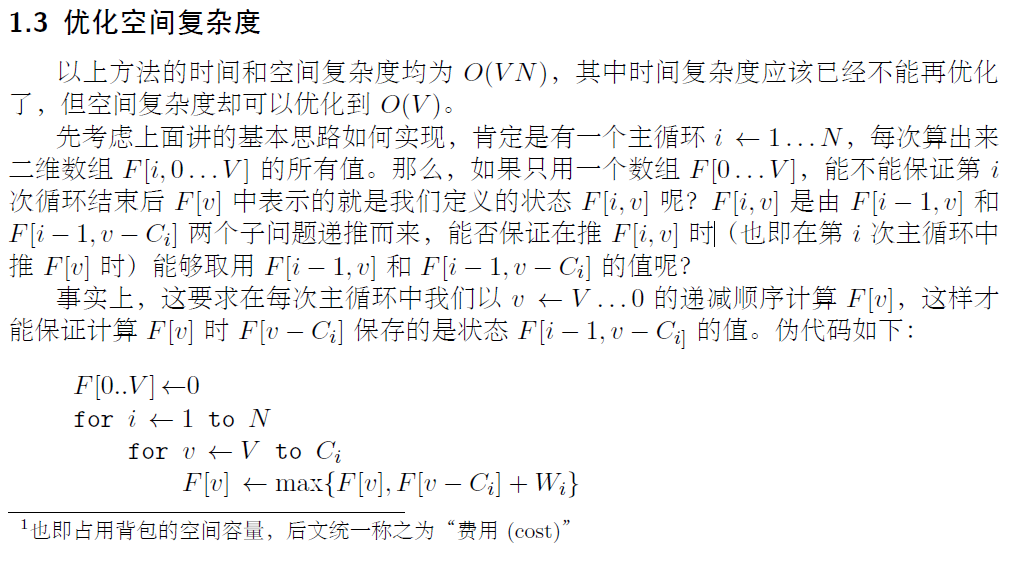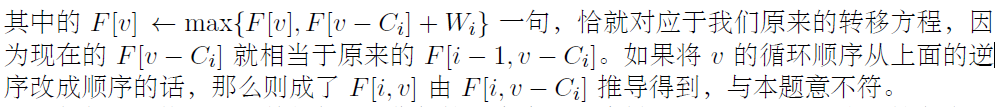### 人话解释：

那么，这里为什么要逆序呢？这是因为，在第i轮还没有开始计算的时候，我们肯定手上已经有了i-1轮的全部结果。而我们的算法理应调用上一轮大循环的小循环整体跑完后算出的结果集来进行运算，也就是每一轮小循环不会需要自己这轮循环里任何的运算数据。

此时我们因为只有一维的数组，所以我们需要考虑的一个问题就是避免状态覆盖。（因为为了节省空间变成每次都修改原数组了）。

即，我不能让新状态，覆盖了老状态，从而导致我原本要用的老状态没了。举例：F(3,4) 需要用 F(2,4) 和 F(2,3) 来计算。（原先二维的时候的状态转移方程的实现代码）。

也就是说，在进行空间的节约操作时，我们需要的思考方向是：如何让我们小循环中每一次先进行的数据更新操作，不会影响到紧随其后的数据更新操作。

综上所述，我们需要让小循环从大往小遍历。

### 最直观的输出过程理解：

``    public static int method2(int[] things, int space, int[] fv) {for (int i = 0; i < things.length; i++) {for (int v = space; v >= 1; v--) {fv[v] = Math.max(fv[v], fv[v - 1] + things[i]);}// 下面三行是输出代码，不是算法内容for (int k = 0; k < fv.length; k++) {System.out.print(fv[k] + "\t");}System.out.println();}return fv[space];}public static void main(String[] args) {int[] things1 = new int[] {1,2,3,4,5,6,7,8};int space1 = 4;int[] fv = new int[space1 + 1];for (int k = 0; k < fv.length; k++) {System.out.print(fv[k] + "\t");}System.out.println();int res2 = method2(things1, space1, fv);System.out.println(res2);}``
``````0	0	0	0	0
0	1	1	1	1
0	2	3	3	3
0	3	5	6	6
0	4	7	9	10
0	5	9	12	14
0	6	11	15	18
0	7	13	18	22
0	8	15	21	26	26``````## 1.4初始化的细节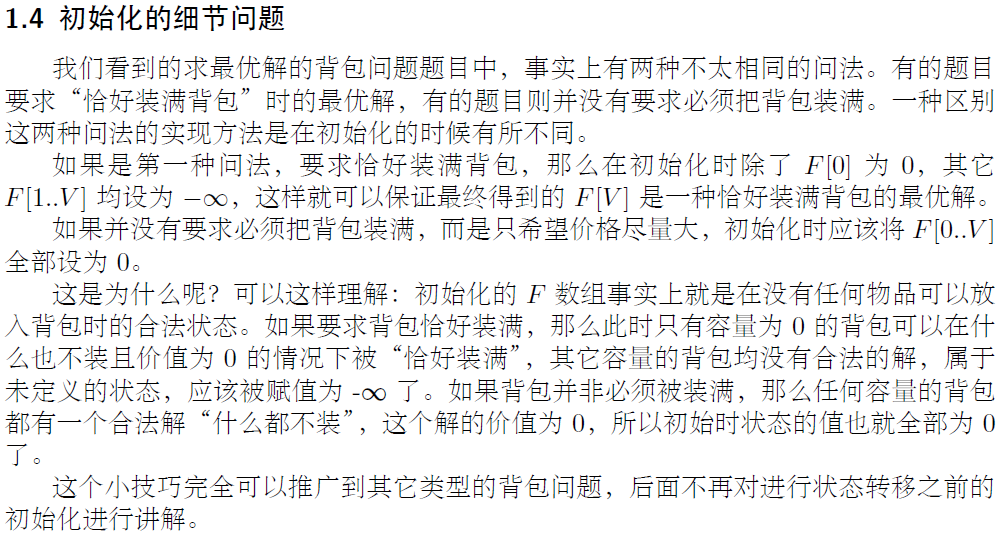### 人话解释：

初始化时的数组要求所有数据状态都是合法的。那么，有如下思考：

如果要求背包恰好装满，此时只有容量为 0 的背包可以装 0 容量合法，0 值对于其余的背包都不是一个合法的值，所以我们用负无穷对其余部分进行初始化。

如果不要求背包必须装满，那么对于任何背包，装零值都是合法的解，所以初始化全零。

（注：上面用来让大家理解的输出代码，写的时候并没有记着遵循这个初始化细节）

## 1.5一个常数的优化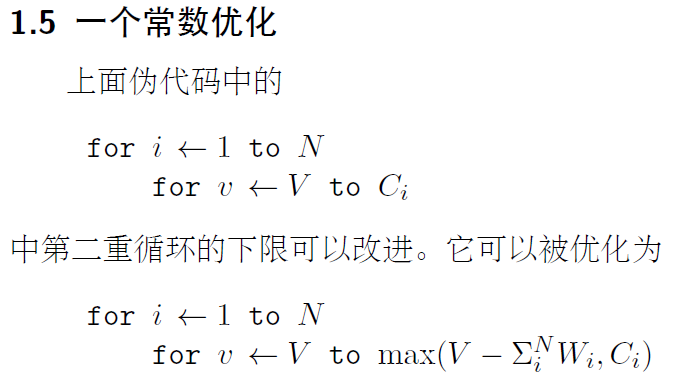### 人话解释：

这是逆序的，所以看见取个 max ，是正常的，因为是让下限扩大。

### 最直观的代码解释：

``    /*** 常数优化*/public static int method3(int[] things, int space, int[] fv) {for (int i = 0; i < things.length; i++) {int temp = 0;for (temp = i + 1; temp < things.length + 1; temp++) {temp += 1;  // 这里的这个 1， 对应的是每个物体的重量。}int bound = Math.max((space - temp), 1);for (int v = space; v >= Math.max(bound, 1); v--) {fv[v] = Math.max(fv[v], fv[v - 1] + things[i]);}// 下面四行是输出代码，不是算法内容for (int k = 0; k < fv.length; k++) {System.out.print(fv[k] + "\t");}System.out.println();}return fv[space];}public static void main(String[] args) {int[] things1 = new int[] {1,2,3,4,5,6,7,8};int space1 = 4;int[] fv = new int[space1 + 1];for (int k = 0; k < fv.length; k++) {System.out.print(fv[k] + "\t");}System.out.println();int res3 = method3(things1, space1, fv);System.out.println(res3);}``
``````0	0	0	0	0
0	1	1	1	1
0	2	3	3	3
0	3	5	6	6
0	4	7	9	10
0	5	9	12	14
0	6	11	15	18
0	7	13	18	22
0	8	15	21	26
26``````

## 1.6小结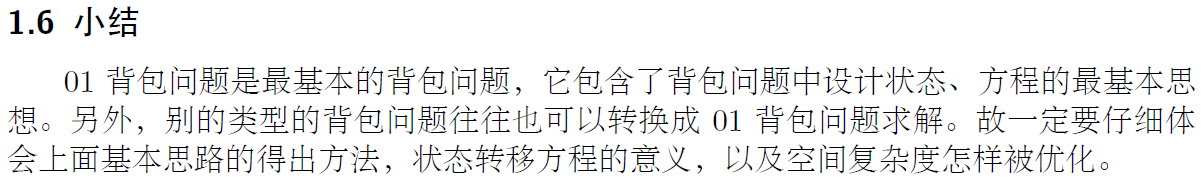今年秋招前，只打算学完完全背包，多重背包我猜面试官不会考我。至于面试官问你多重背包怎么办？你告诉他，把多重背包拆成0/1背包，然后按0/1背包写，写完后再和他商量要不要优化。

## 2.1基本思路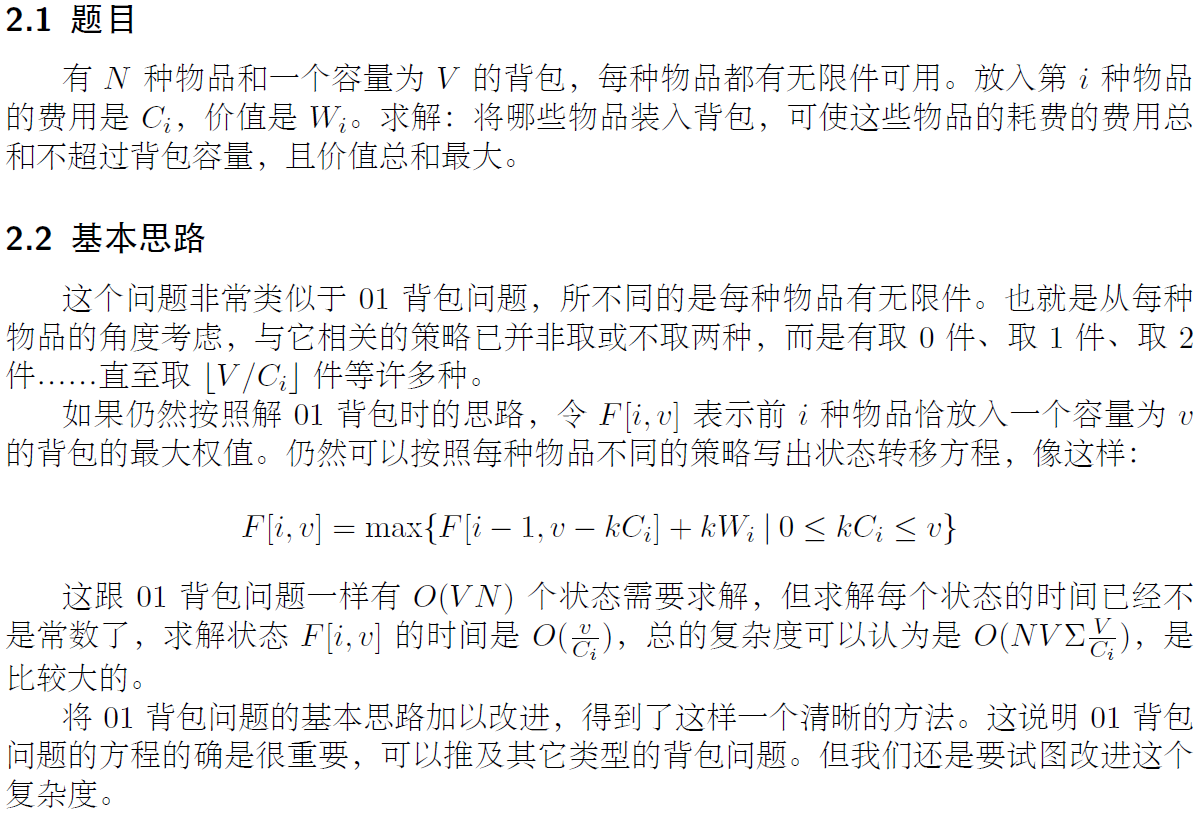### 人话解释：

这个状态转移方程就是说 F(i) 表示：选择在取 k 个 物品 i，且 容量为 v - k*Ci 的情况下 取前 i - 1 个物品的最优解。

取 k 个物品 i， 会导致我们的可用容量减少 k * Ci 这么多，我们的总容量是 v ， 那么就是用 v - k*Ci 的容量，去取前 i - 1种不同的物品。（在我们算 i 的时候， 前 i - 1 种物品所对应的任意容量的状态肯定是已知的。）

### 最直观的代码解释：

``````// 问题描述：有N 种物品和一个容量为V 的背包，每种物品都有无限件可用。放入第i 种物品// 的费用是Ci，价值是Wi。求解：将哪些物品装入背包，可使这些物品的耗费的费用总// 和不超过背包容量，且价值总和最大。// 1、F[i,v] = max{F[i - 1, v - kCi] + kWi | 0 <= kCi <= v}// 假如我们用 f[i][j]表示前i件物品放入一个容量为j的背包的最大价值。具体的状态转移方程如下// f[i][j] = max{f[i-1][j-k*w[i]] + k*v[i] | 0 <= k*w[i] <= j} (我们的数组应该记录着 0 <= j <= V)的全部状态// k为物品i我们选择放k个进背包。//// 编写一个函数来计算可以凑成总金额所需的最少的硬币个数。如果没有任何一种硬币组合能组成总金额，返回 -1。/**** @param v v[i] 是第i件物品的价值* @param V V是需要计算的背包的容量* @param w w[i] 是第i件物品的重量* @return*/public int coinChange(int[] v, int V, int[] w) {int vLength = V + 1;int[][] f = new int[v.length][vLength];// f[i]for (int i = 0; i < v.length; i++) {// f[i][j]for (int j = 0; j < vLength; j++) { // j 最多可以加到Vif (i == 0) {int k = j / w[i];f[i][j] = 0 + k * v[i];} else {int k = j / w[i];int max = 0;// max{f[i-1][j-k*w[i]] + k*v[i] | 0 <= k*w[i] <= j} // 下面这个循环是用来取 max 的。for (int a = 0; a <= k; a++) {if(f[i - 1][j - a * w[i]] + a * v[i] > max) {max = f[i - 1][j - a * w[i]] + a * v[i];}}f[i][j] = max;}}}System.out.println();System.out.println("f数组最后结果如下：");for (int i = 0; i < f.length; i++) {for (int j = 0; j < f.length; j++) {System.out.printf("%d\t", f[i][j]);}System.out.println();}return 0;}public static void main(String[] args) {// 1、测试数据1
//        int[] v = new int[]{3,8,20};
//        int[] w = new int[]{3,5,10};
//        int V = 13;// 2、测试数据2
//        int[] v = new int[]{0,8,20};
//        int[] w = new int[]{3,5,10};
//        int V = 13;// 3、测试数据3
//        int[] v = new int[]{1,8,20};
//        int[] w = new int[]{1,5,10};
//        int V = 13;// 4、测试数据4int[] v = new int[]{3,8,10};int[] w = new int[]{2,5,10};int V = 13;new Theory().coinChange(v, V, w);}``````

## 2.3第一种简单的优化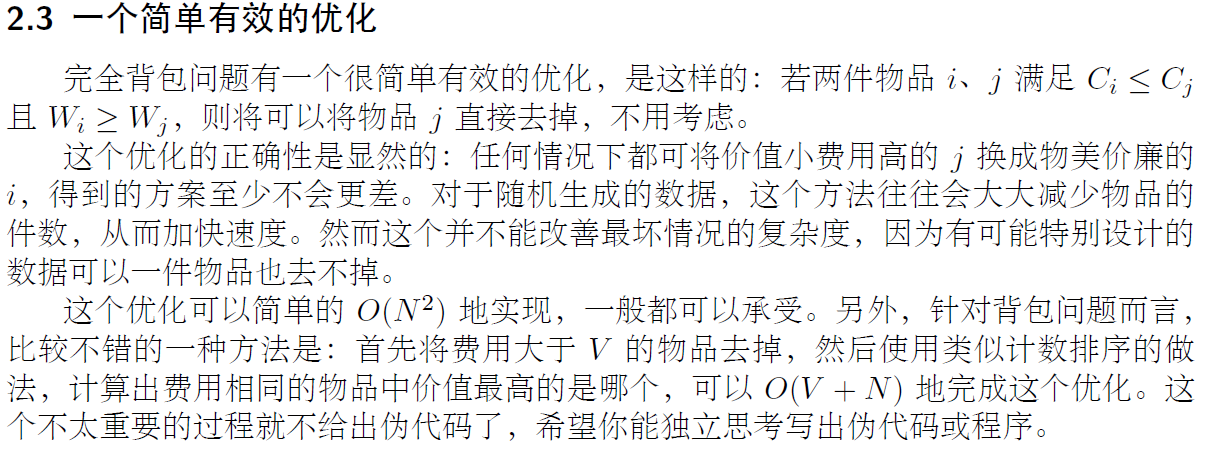## 2.4转化为0/1背包求解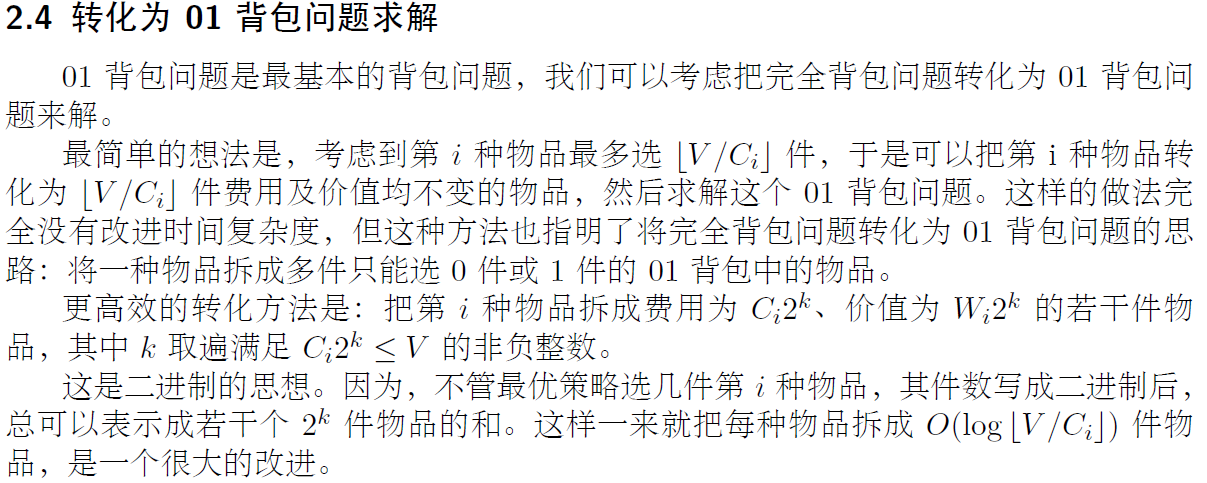## 2.5 O(VN)的算法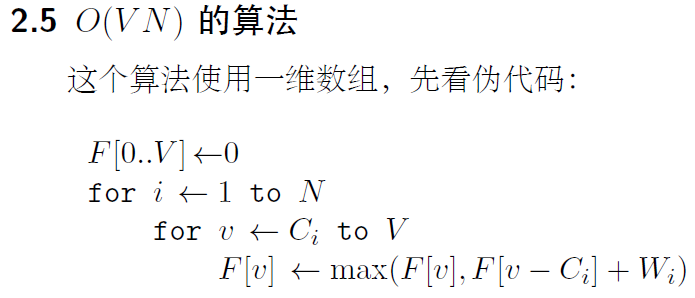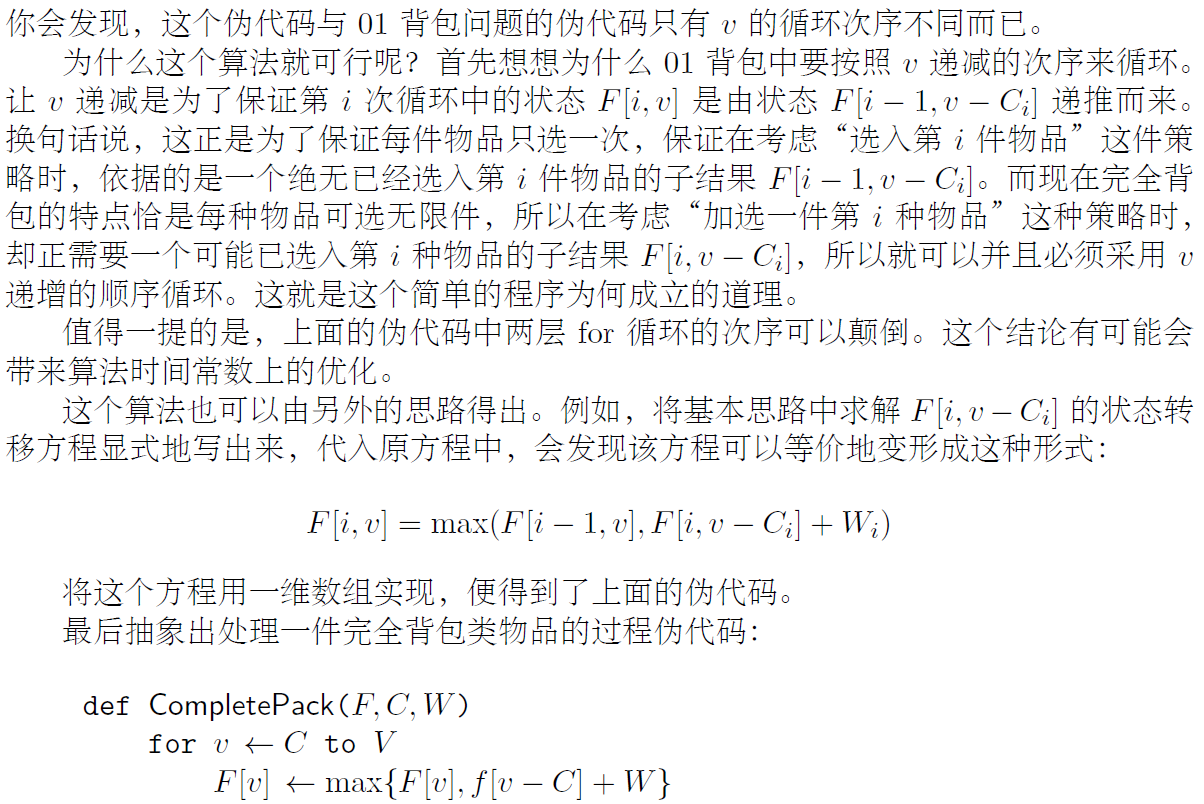### 2.6小结